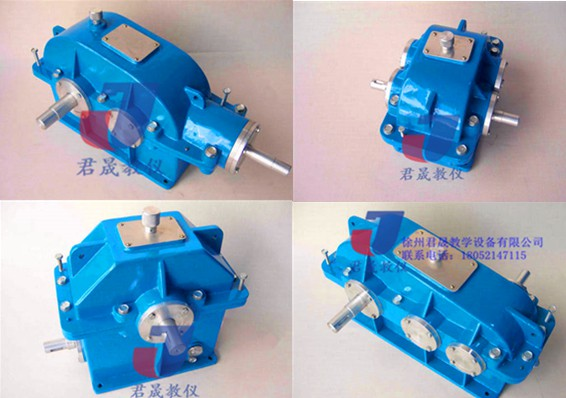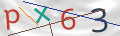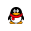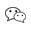18052147115

JS-CM型 拆装用大型减速器（全铝制）
2018-11-23 17:28:45
2590次访问

### 南通液压实训装置厂家•1、 了解铸造箱体的结构；
2、 观察、了解减速器附属零件的用途、结构和安装位置的要求；
3、测量减速器的中心距、中心高、箱座上、下凸缘的宽度和厚度、筋板的厚度、齿轮端面（蜗轮轮毂）与厢体内壁的距离、大齿轮顶圆（蜗轮外圆）与箱内壁之间的距离、轴承内端面至箱内壁之间的距离等；
4、观察了解蜗杆减速器厢体侧面（蜗轮轴向）宽度与蜗杆的轴承盖外圆之间的关系。为提高蜗杆轴的刚度，仔细观察蜗杆轴的结构特点；
5、了解轴承的润滑方式和密封位置，包括密封的形式。轴承内侧挡油环、封油环的作用原理及其结构和安装位置；
6、了解轴承的组合结构以及轴承的拆装、固定和轴向间隙的调整；测绘轴系部件的结构图。

 编号 名  称 单位 数量 参数及尺寸 备注 1 JS-C1型拆装用双级同轴式圆柱齿轮减速器 台 1 m=3  Z1=22  Z2=26   Z3=78    Z4=82 ɑ=20°β=15°  i=11.1818  h=159mm      外形尺寸:560㎜×420㎜×350㎜ 重量:24.8KG 全铝制 2 JS-C2型拆装用双级展开式圆柱齿轮减速器 台 1 m=3   Z1=16   Z2=47  Z3=27  Z4=68 а1=99 ɑ=20°β=15°  i=7.398  h=146mm   外形尺寸:535㎜×330㎜×294㎜  重量:21KG 全铝制 3 JS-C3型拆装用双级圆锥圆柱齿轮减速器 台 1 m=3  Z1=20 Z2=50 Z3=16   Z4=68  i=10.625  h=141mm  a=130.5 β=15°外形尺寸:550㎜×460㎜×360㎜  重量:20KG 全铝制 4 JS-C 4型拆装用单级圆锥齿轮减速器 台 1 m=3  Z1=20  Z2=60ɑ=20°β15°i=3  h=129mm外形尺寸:500×350×315     重量:15.5KG 全铝制 5 JS-C 5型拆装用单级圆柱齿轮减速器 台 1 m=3  Z1=72  Z2=29 ɑ=20°β=15°a=158    i=3  h=151mm外形尺寸:450㎜×400㎜×340㎜  重量:17.5KG 全铝制 6 JS-C 6型拆装用蜗轮蜗杆减速器 台 1 m=4 z1=1  z2=45 β=15° i=45   H=163外形尺寸：420㎜×320㎜×400㎜  重量   15.5KG 全铝制 7 JS-C 7型拆装用新型结构单级圆柱齿轮减速器 台 1 m=2  Z1=25  Z2=75   i=3   h=120外形尺寸：290㎜×230㎜×220㎜ 全铝制 8 JS-C 8型拆装用双级分流式圆柱齿轮减速器 台 1 m=3  Z1=16  Z2=47  Z3=27  Z4=68       ɑ=20°  β=15°   i=7.398      h=146mm外形尺寸:540㎜×360㎜×320㎜  重量:24.5KG 全铝制18052147115